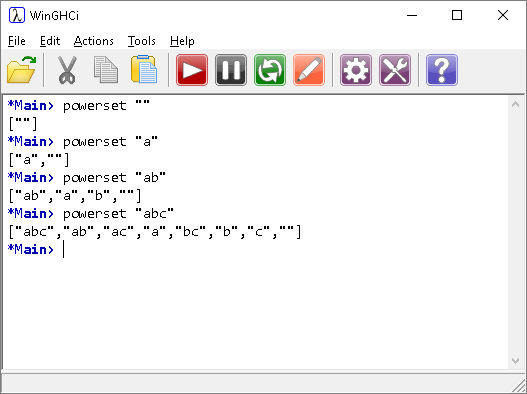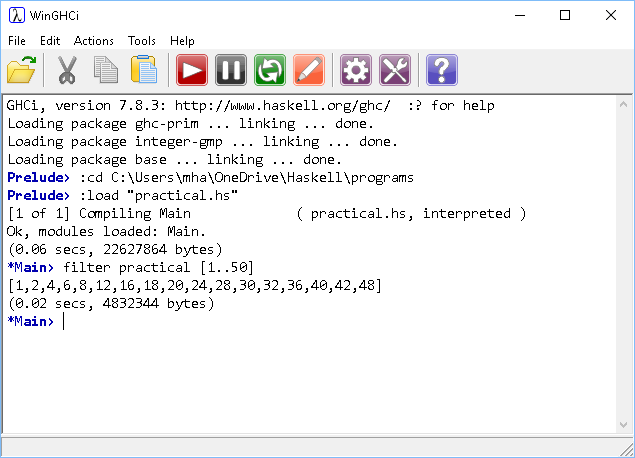# Introduction To Haskell Power Sets & Practical Numbers

## Introduction

The power set of a set S is a set of all of the subsets of S, including the empty set and S itself. It is quite an easy thing to express in Haskell and a function that I find myself using quite often when solving certain problems.

To find out if a number is a practical number, first work out its proper divisors. The proper divisors of a number are all of its factors except itself. The proper divisors of 6 are,

1, 2, 3

Then work out all of the possible ways that you could add these factors together. For the factors of 6, this would be,

• 1
• 2
• 3
• 1 + 2 = 3
• 1 + 3 = 4
• 2 + 3 = 5
• 1 + 2 + 3 = 6

If all of the numbers up to the number can be found by adding together combinations of factors, then the number is a practical number. 6 is a practical number because the digits, 1, 2, 3, 4 and 5 appear in this list.

Take the number 5. It is a prime number, so its only proper divisor is 1. 1 is the only digit that can be made from the factors and so 5 is not a practical number.

Generating this sequence is included here because of its dependence on the power set of the factor list.

## Programming - Power Set

This is the power set function.

```powerset [] = [[]]
powerset (x:xs) = map (x:) (powerset xs) ++ powerset xs
```

The first line says that the powerset of an empty set is an empty set. Our function is meant to return a list of lists.

The second line is where the magic happens. The (x:xs) is pattern matching. Using this with a list binds x to the head of the list and xs to the tail of the list.

This is worth testing out a little to get a better understanding of how it works.Now, taking the first example, we''ll start with an empty list and the increase one item at a time.## Programming - Practical Numbers

Despite the apparent complexity of the problem, it is relatively easy to break down these steps in Haskell.

```powerset [] = [[]]
powerset (x:xs) = map (x:) (powerset xs) ++ powerset xs

pdivs p = [x|x<-[1..div p 2],mod p x==0]

summings p = map (sum) (powerset (pdivs p))

practical p = all (`elem` (summings p)) [1..p-1]
```

The pdivs function uses a list comprehension to give the proper divisors of the input. Summings takes the powerset of the proper divisors and maps the sum function to give a list of the totals that can be made of these divisors. The final function tests whether a number p is practical using the all function to see if the preceeding integers are all present in the summings list.## Challenge

Although this performs quite well, it could be a little more efficient. Apart from 1 and 2, all practical numbers are divisible by either 4, 6 or both.